# Basic Arithmetic : Division with Fractions

## Example Questions

← Previous 1

### Example Question #11 : Fractions

Solve the following: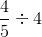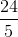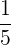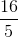Explanation:

The correct answer isand can be found by using reciprocals as shown below: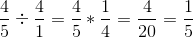The first step is to identify that 4 can be written as 4 divided by 1. You can then take the first fraction and multiple it by the reciprocal of the second fraction, which can be found by switching the numerator and denominator. From there, you solve and simplify until you get to the final answer.

### Example Question #1 : Division With Fractions

Evaluate the following division problem and express the resulting fraction in its simplest form.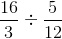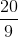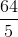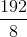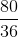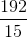Explanation:

In order to divide two fractions you simply find the inverse of either fraction, and multiply that by the other fraction.

To find the inverse of a fraction, you just switch the numerator and the denominator.

The inverse of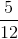is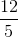.

So to divide:We turn it into the following multiplication problem: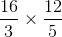We multiply the numerators by each other and the denominators by each other to carry out the multiplication: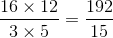Both 192 and 15 are divisible by 3, so we divide 192 by 3 and 15 by 3 and are left with:### Example Question #1 : Division With Fractions

Determine the answer as an improper fraction: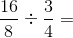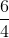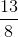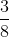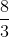Explanation:

1. Invert the second fraction: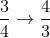2. Multiply the two fractions: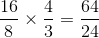3. Reduce the improper fraction: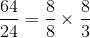So the improper fraction can be reduced to.

### Example Question #11 : Fractions

What is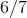divided by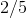?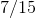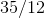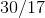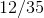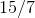Explanation:

To divide fractions, simply invert the second fraction and multiply.  So,divided bybecomes: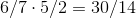reduce to find the correct answer:.

### Example Question #1 : Multiplying And Dividing Fractions

Simplify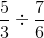.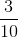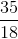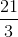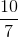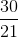Explanation:

To solve, we must turn the division problem into a multiplication problem by "flipping" the second fraction (dividing by a fraction is the same as multiplying by its reciprocal):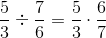.

Then, we multiply the numerators followed by the denomenators: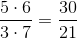.

Lastly, the fraction must be simplified by a factor of 3: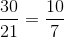, which gives us our final answer.

### Example Question #21 : Operations With Fractions

Evaluate: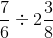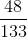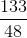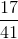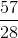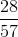Explanation:

Change all the fractions to improper fractions.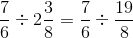Convert the division sign to a multiplication sign, but flip the second term.  Simplify.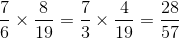### Example Question #1 : Division With Fractions

Divide and simplify: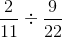.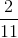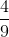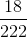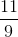Explanation:

Multiplying fractions is a two-step process. First, you must flip the second fraction (make it its reciprocal) and then set up the equation as a multiplication problem: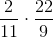.

Then, cross-reduce: 11 goes into both 11 and 22, so you can take that out so it looks like: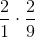.

Then, multiply straight across so that you get.

### Example Question #1 : Division With Fractions

Divide these fractions: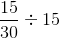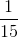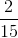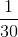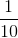Explanation:

When dividing fractions, first we need to flip the second fraction. Then multiply the numerators together and multiply the denominators together: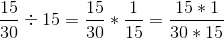Simplify the fraction to get the final answer: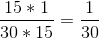### Example Question #1 : Division With Fractions

Divide these fractions: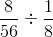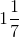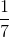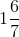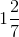Explanation:

When dividing fractions, first we need to flip the second fraction. Then multiply the numerators together and multiply the denominators together: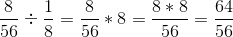Simplify the fraction to get the final answer: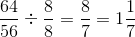### Example Question #21 : Fractions

Divide these fractions: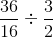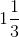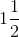Explanation:

When dividing fractions, first we need to flip the second fraction. Then multiply the numerators together and multiply the denominators together: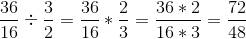Simplify the fraction to get the final answer: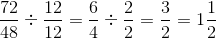← Previous 1

### All Basic Arithmetic Resources